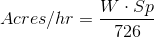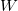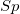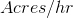# Traveling Sprinkler

This calculator finds the number of irrigated acres per hour, based on lane spacing, and travel speed. Lane spacing is the distance between the pulls on a traveling sprinkler. The travel speed is the rate that the traveling sprinkler is reeled in. Learn more about the units used on this page.

Lane Spacing:
Travel Speed:
Total Irrigated Acres per Hour:
acres/hr

## The Equation

This calculator uses this formula to determine the area irrigated per hour by a traveling sprinkler.Where:= The width between pulls of the traveling sprinkler (ft)= The speed that the traveling sprinkler is reeled in (ft/min)= Total irrigated area per hour (acres/hr)

WSU Prosser - IAREC, 24106 N Bunn Rd, Prosser WA 99350-8694, 509-786-2226. Contact Us